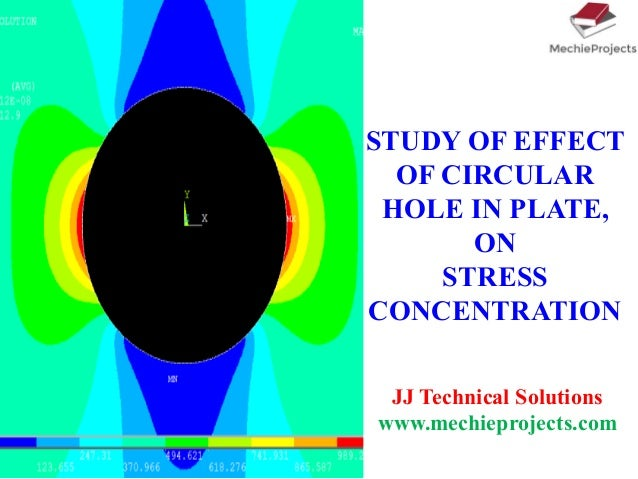Successfully reported this slideshow.Upcoming SlideShare
×

Effect of Hole on Stresses of a Flat Plate

2,057 views

Published on

Study of Effect of Circular Hole in Flat Plate on Stresses. the stresss distribution is studied through Finite element analysis Ansys

Published in: Engineering
• Full Name
Comment goes here.

Are you sure you want to Yes No• For more free projects and complete presentations visit www.mechieprojects.com

Are you sure you want to  Yes  No
• Be the first to like this

Effect of Hole on Stresses of a Flat Plate

1. 1. STUDY OF EFFECT OF CIRCULAR HOLE IN PLATE, ON STRESS CONCENTRATION JJ Technical Solutions www.mechieprojects.com
2. 2. AIM • To study the stresses in flat plate with circular hole, for different pressure loads (P=50 MPa to 450 MPa) • To study the effect of increase in pressure on the max. stresses developed. • To study the stress profile across the circular hole, through the plate width. • To tabulate and plot the max. stress generated in the flat plate with circular hole for different pressure loads.
3. 3. SOLVER: ANSYS 14.0 MESH: STRUCTURAL SOLID “PLANE 182”; (QUAD 4 NODE 182) SOLUTION: STEADY STATE STRUCTURAL SOLUTION MATERIAL: Al. ALLOY EX: 69E9 Pa PRXY: 0.3 No. OF ELEMENTS: 244000 BOUNDARY CONDITIONS FOR THE PROBLEM STATEMENT P = 1. 50 MPa 2. 150 MPa 3. 250 MPa 4. 350 MPa 5. 450 MPa ϕ0.04 0.2 0.4 P Fixed Edge of Plate Units: Length: m Pressure: MPa Stress: MPa
4. 4. STRUCTURED MESH ELEMENTS IN THE PLATE – SMOOTH MESHING No. OF ELEMENTS: 244000 (Typ.) MESH: STRUCTURAL SOLID “PLANE 182”; (QUAD 4 NODE 182)
5. 5. PRINCIPAL STRESS PLOT IN THE PLATE FOR σ = 50 MPa σ = 50 MPa
6. 6. PRINCIPAL STRESS PLOT (NEAR THE HOLE)IN THE PLATE FOR σ = 50 MPa
7. 7. 0 0.08 σ = 50 MPa
8. 8. PRINCIPAL STRESS PLOT IN THE PLATE FOR σ = 150 MPa σ = 150 MPa
9. 9. PRINCIPAL STRESS PLOT (NEAR THE HOLE)IN THE PLATE FOR σ = 150 MPa
10. 10. 0 0.08 σ = 150 MPa
11. 11. PRINCIPAL STRESS PLOT IN THE PLATE FOR σ = 250 MPa σ = 250 MPa
12. 12. PRINCIPAL STRESS PLOT (NEAR THE HOLE)IN THE PLATE FOR σ = 250 MPa
13. 13. 0 0.08 σ = 250 MPa
14. 14. PRINCIPAL STRESS PLOT (NEAR THE HOLE)IN THE PLATE FOR σ = 350 MPa
15. 15. 0 0.08 σ = 350 MPa
16. 16. PRINCIPAL STRESS PLOT IN THE PLATE FOR σ = 450 MPa σ = 450 MPa
17. 17. PRINCIPAL STRESS PLOT (NEAR THE HOLE)IN THE PLATE FOR σ = 450 MPa
18. 18. 0 0.08 σ = 450 MPa
19. 19. 0 0.08 VM: Von-Misses 1st Principal: Principal Stress
20. 20. (MPa) Linear Correlation between Pressure and Max. Stress
21. 21. Results and Discussions • The max. stresses generated due to various pressure loads on the flat plate configuration are: • The max. stress generated is a linear function of Pr. Load. • The stress distribution across the width of the plate is plotted • The stress distribution across the width of the plate for different Pr. Loads are plotted and studied. • The stress distribution around the hole is studied. Sl.No. Pressure (MPa) Max. Stress (MPa) 1 50 159 2 150 477 3 250 795 4 350 1113 5 450 1431
22. 22. FOR MORE PROJECTS PRESENTATIONS AND PROJECT REPORTS VISIT WWW.MECHIEPROJECTS.COM THANKYOU